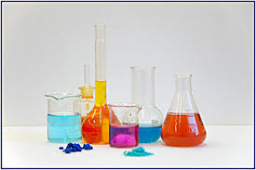# Kilograms 63624

How many kg of iron and how many kg of sulfur does 100 kilograms of iron sulfide (FeS) contain if the relative atomic weight of iron is 52 and sulfur 32?

m1 =  61.9 kg
m2 =  38.1 kg

### Step-by-step explanation:Did you find an error or inaccuracy? Feel free to write us. Thank you!

Tips for related online calculators
Check out our ratio calculator.
Do you have a system of equations and looking for calculator system of linear equations?